# 23. Standard process inputs¶

:

import sympy
import matplotlib.pyplot as plt
sympy.init_printing()
%matplotlib inline


## 23.1. Step¶

A step input of magnitude $$M$$ can be written as

$\begin{split}u_S(t)=\begin{cases} 0& t<0,\\ M& t\geq 0 \end{cases}\end{split}$

Sympy supplies a unit step function called Heaviside, which is typeset as $$\theta(t)$$

:

t = sympy.symbols('t')

:

S = sympy.Heaviside

:

M = 2

:

sympy.plot(M*S(t))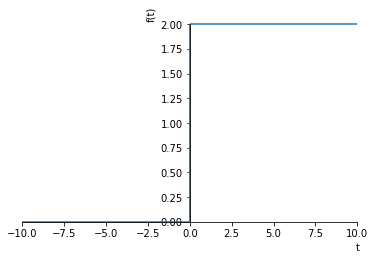:

<sympy.plotting.plot.Plot at 0x1178f46a0>


## 23.2. Laplace transform¶

Sympy can calculate laplace transforms of the step easily:

:

M, s = sympy.symbols('M, s')

:

def L(f):
return sympy.laplace_transform(f, t, s, noconds=True)
def invL(F):
return sympy.inverse_laplace_transform(F, s, t)

:

L(M*S(t))

:

$$\frac{M}{s}$$

## 23.3. Scaling and translation¶

We can scale and translate the step function in the normal way. Notice that how the time translation is handled.

:

from ipywidgets import interact

:

def translated_step(scale, y_translation, t_translation):
f = scale*S(t - t_translation) + y_translation
print("f =", f, "  \u2112(f) =", L(f))
sympy.plot(f, (t, -10, 10), ylim=(-2, 4))

:

interact(translated_step,
scale=(0.5, 3.),
y_translation=(-1., 1.),
t_translation=(0., 5.));


## 23.4. Rectangular pulse¶

It is now easy to see how we can construct a rectangular pulse, of height $$h$$ and width $$t_w$$,

$\begin{split}u_{RP}(t)=\begin{cases} 0& t<0,\\ h& 0 \leq t < t_w\\ 0& t \geq t_w \end{cases}\end{split}$

by using shifted versions of the step, so that

:

h, t_w = sympy.symbols('h, t_w')
u_RP = h*(S(t - 0) - S(t - t_w))

:

sympy.plot(u_RP.subs({h: 1, t_w: 2}), (t, -1, 3));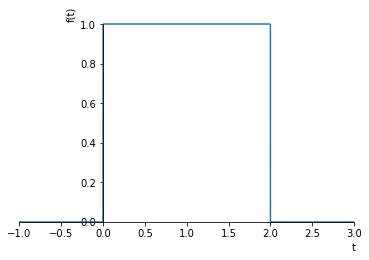:

L(u_RP)

:

$$\frac{h}{s} - \frac{h}{s} e^{- s t_{w}}$$

### 23.4.1. Arbitrary piecewise constant functions¶

We can constuct any piecewise constant function by adding together step functions shifted in time. As an example, we can take the function represented below:

:

x = [-1, 0, 0, 1, 1, 2, 2, 3]
y = [0, 0, 1, 1, 2, 2, 0, 0]

:

plt.plot(x, y)
plt.text(0, 0.5, r'$\Delta_1=1$')
plt.text(1, 1.5, r'$\Delta_2=1$')
plt.text(2, 1, r'$\Delta_3=-2$')

plt.text(0, 0.1, r'$D_1=0$')
plt.text(1, 0.1, r'$D_2=1$')
plt.text(2, 0.1, r'$D_3=2$')

:

Text(2,0.1,'$D_3=2$')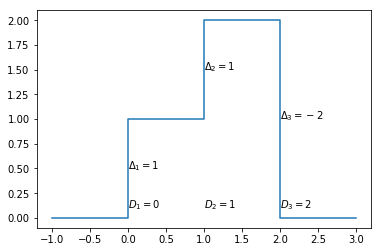In general piecewise constant functions like the one above can be written as

$f_c(t) = \sum_{i=1}^{N_d} \Delta_i S(t - D_i)$

where $$S$$ is the unit step. We calculate $$\Delta_i$$ as the difference between the values at the discontinuities, positive if the function is rising and negative if it is falling. $$D_i$$ is the time at which the value changes and $$N_d$$ is the number of discontinuities.

We can apply this directly for the example function.

:

f = 1*S(t) + 1*S(t-1) - 2*S(t-2)
f

:

$$\theta\left(t\right) - 2 \theta\left(t - 2\right) + \theta\left(t - 1\right)$$

Or a little more generally using some code:

:

Delta = [1, 1, -2]
D = [0, 1, 2]
Nd = len(Delta)

:

f = sum(Delta[i]*S(t - D[i]) for i in range(Nd))

:

f

:

$$\theta\left(t\right) - 2 \theta\left(t - 2\right) + \theta\left(t - 1\right)$$

Let’s verify it works properly:

:

sympy.plot(f, (t, -1, 3));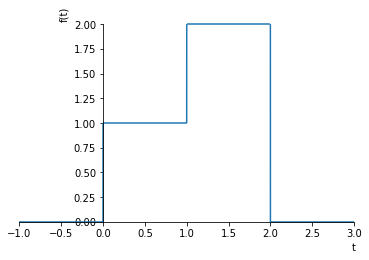:

sympy.expand(L(f))

:

$$\frac{1}{s} + \frac{e^{- s}}{s} - \frac{2}{s} e^{- 2 s}$$

## 23.5. Ramp¶

A ramp with slope $$a$$ can be written as

$\begin{split}u_R(t)=\begin{cases} 0& t<0,\\ a& t\geq 0 \end{cases}\end{split}$

We can construct a unit ($$a=1$$) ramp by simply multiplying the unit step by $$t$$

:

def R(t):
return t*S(t)

:

sympy.plot(R(t))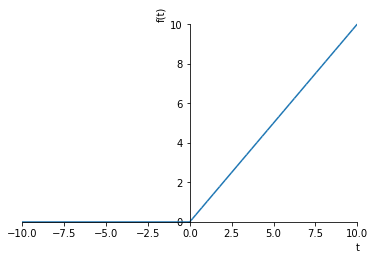:

<sympy.plotting.plot.Plot at 0x11a539198>

:

L(R(t))

:

$$\frac{1}{s^{2}}$$

## 23.6. Continuous piecewise linear functions¶

We can build any continuous piecewise linear function by using shifted and scaled ramps.

$f(t) = \sum_{i=1}^{N_s} \Delta m_i R(t - D_{s,i})$

This time $$\Delta m_i$$ represents changes in slopes ($$m=\frac{\Delta y}{\Delta x}$$). $$D_{s,i}$$ are the times at which the slopes change and $$N_s$$ is the number of slope changes.

For instance, we can construct a triangular pulse by adding three ramps together.

:

r1 = t*S(t - 0)
r2 = -2*(t - t_w/2)*S(t - t_w/2)
r3 = (t - t_w)*S(t - t_w)
u_TP = 2/t_w*(r1 + r2 + r3)

:

u_TP

:

$$\frac{2}{t_{w}} \left(t \theta\left(t\right) + \left(- 2 t + t_{w}\right) \theta\left(t - \frac{t_{w}}{2}\right) + \left(t - t_{w}\right) \theta\left(t - t_{w}\right)\right)$$
:

sympy.plot(u_TP.subs({t_w: 2}), (t, -1, 4))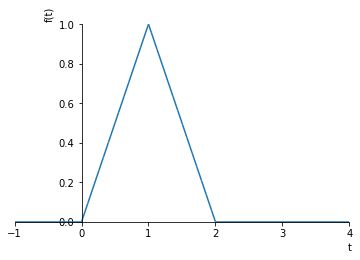:

<sympy.plotting.plot.Plot at 0x11a6de550>

:

L(u_TP.subs({t_w: 2})).expand()

:

$$\frac{1}{s^{2}} - \frac{2}{s^{2}} e^{- s} + \frac{1}{s^{2}} e^{- 2 s}$$

Notice that there are three ramps here (one may have expected only two). It becomes more clear when we think about the derivative of this function:

:

sympy.plot(u_TP.diff(t).subs({t_w: 2}), (t, -1, 4), ylim=(-1.1, 1.1))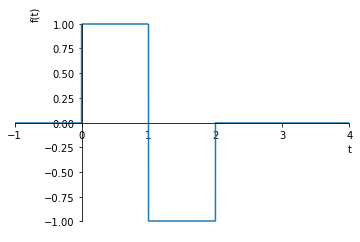:

<sympy.plotting.plot.Plot at 0x11c5a5710>


We now see that the derivative of a piecewise linear continuous function is a piecewise constant function. We can apply our rule for piecewise constant functions and integrate the steps to ramps:

:

derivative = 1*S(t-0) - 2*S(t-1) + 1*S(t-2)

:

final = 1*R(t-0) - 2*R(t-1) + 1*R(t-2)


## 23.7. Arbitrary piecewise linear functions¶

We can construct any piecewise linear function by adding together ramp functions and steps shifted in time. The general rule now becomes

$f(t) = \underbrace{\sum_{i=1}^{N_s} \Delta m_i R(t - D_{s,i})}_\text{slope changes} + \underbrace{\sum_{i=1}^{N_d} \Delta_i S(t - D_i)}_\text{discontinuities}$
:

x = [-1, 0, 2, 2, 3, 4, 5]
y = [0, 0, 1, 2, 2, 0, 0]

:

plt.plot(x, y)

:

[<matplotlib.lines.Line2D at 0x11ac04d30>]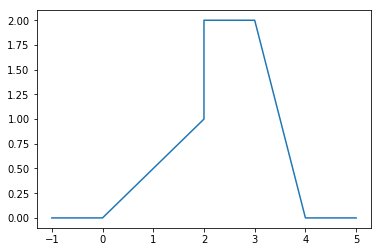We see that there are 4 slope changes (at t=0, t=2, t=3 and t=4) and 1 discontinuity. Applying our formula yields:

:

g = 0.5*R(t) - 0.5*R(t-2) - 2*R(t-3) + 2*R(t - 4) + S(t - 2)

:

sympy.plot(g, (t, -1, 5));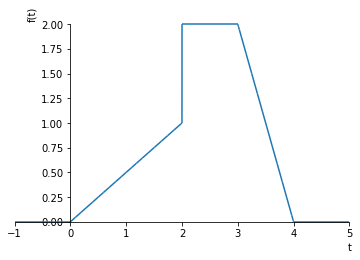:

sympy.expand(L(g))

:

$$\frac{1.0}{s} e^{- 2 s} + \frac{0.5}{s^{2}} - \frac{0.5}{s^{2}} e^{- 2 s} - \frac{2.0}{s^{2}} e^{- 3 s} + \frac{2.0}{s^{2}} e^{- 4 s}$$